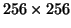Next: Example: Reading with dimension Up: Image conversion variables Previous: Example: Writing values   Contents

### Dimension conversion

One of the problems of arbitrary dimensioned images is that it becomes necessary for software to handle the general case. It is easier to write application software if it is known in advance that all images will have a specific size (e.g.) and a specific orientation (e.g. the first pixel is at the patient's anterior, right side).

By setting the icv property MI_ICV_DO_DIM_CONV to TRUE these conversions can be done automatically. The orientation of spatial axes is determined by the properties MI_ICV_XDIM_DIR, MI_ICV_YDIM_DIR and MI_ICV_ZDIM_DIR. These affect any image dimensions that are MI?space or MI?frequency where ? corresponds to x, y or z. These properties can have values MI_ICV_POSITIVE, MI_ICV_NEGATIVE or MI_ICV_ANYDIR. The last of these will prevent flipping of the dimension. The first two will flip the dimension if necessary so that the attribute MIstep of the dimension variable will have the correct sign.

The two image dimensions are referred to as dimensions A and B. Dimension A is the fastest varying dimension of the two. Setting properties MI_ICV_ADIM_SIZE and MI_ICV_BDIM_SIZE specify the desired size for the image dimension. Dimensions are resized so that the file image will fit entirely in the calling program's image, and is centred in the image. The size MI_ICV_ANYSIZE allows one of the dimensions to have a variable size. If property MI_ICV_KEEP_ASPECT is set to TRUE, then the two dimensions are rescaled by the same amount. It is possible to inquire about the new step and start, corresponding to attributes MIstep and MIstart (where pixel position = ipixel*step + start, with ipixel counting from zero). The properties MI_ICV_?DIM_STEP and MI_ICV_?DIM_START (? = A or B) are set automatically and can be inquired but not set.

Although vector images are allowed, many applications would rather only deal with scalar images (one intensity value at each point). Setting MI_ICV_DO_SCALAR to TRUE (the default) will cause vector images to be converted to scalar images by averaging the components. (Thus, RGB images are automatically converted to gray-scale images in this simple way).

It can sometimes be useful for a program to perform dimension conversions on three (or perhaps more) dimensions, not just the two standard image dimensions. To perform dimension flipping and/or resizing on dimensions beyond the usual two, the property MI_ICV_NUM_IMGDIMS can be set to an integer value between one and MI_MAX_IMGDIMS. To set the size of a dimension, set the property MI_ICV_DIM_SIZE (analogous to MI_ICV_ADIM_SIZE). To specify the dimension to be set, add the dimension to the property (adding zero corresponds to the fastest varying dimension -- add zero for the A'' dimension, one for the B'' dimension, etc.). Voxel separation and location can be inquired about through the properties MI_ICV_DIM_STEP and MI_ICV_DIM_START (analogous to MI_ICV_ADIM_STEP and MI_ICV_ADIM_START), again adding the dimension number to the property.Next: Example: Reading with dimension Up: Image conversion variables Previous: Example: Writing values   Contents
Robert VINCENT 2004-05-28